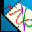# MATHPLOT(1)

english, spanish## NAME

mathplot, mathplot2ps - interactive (or batch) function grapher

## SYNOPSIS

mathplot [-h | --help] [-f file] [functions...]
mathplot2ps [-h | --help] [-f file] [functions...]

## DESCRIPTION

mathplot is a GUI frontend for interactive graphing of functions. mathplot2ps is a batch program to generate PostScript output.

You can have both equations (y=f(x) or f(x,y)=g(x)) and inequations. For inequations, mathplot hatches the intersection. For example, with y>0, y<x and x<2 it hatches the solution which is a triangle.
You can name a point, anywhere or on a function. You can ask for the tangent of a function.
As it uses a symbolic library, you can enter functions like "x+1/y=2".
Can find root (zeros), extrema (even symbolically, sometimes) and intersection of 2 functions.
mathplot exports in PostScript so that you can print easily, and with the reference mark (O, i, j).
You can save and load files. mathplot2ps can transform these files into PostScript.
It's written in OCaml using the Tk toolkit for the GUI frontend

## OPTIONS

"-h, --help" Show the usage.

"-f file" Reads the different objects to draw from the file

"functions..." Functions to plot

## SHORTCUTS

For most menu commands corresponds a keystroke. You can see them in the menus. For the buttons, here are the shortcuts:
```
Ctl+Arrows  move the range to the corresponding direction
Ctl+-       zoom out
Ctl++       zoom in
```

gnuplot(1), geg(1).

## AUTHOR

Pixel (Pascal Rigaux) <pixel@rigaux.org>,

Thanks to Jérome Marant for the cute icons :)

## UNRESTRICTIONS

mathplot is Open Source; anyone may redistribute copies of mathplot to anyone under the terms stated in the GNU General Public License.

You can find mathplot at
http://rigaux.org/mathplot-0.8.5.src.tar.gz and
http://rigaux.org/mathplot-0.8.5.bin.i386.dynamic.tar.gz.

This man page is online at
http://rigaux.org/mathplot.html, with screenshots
http://rigaux.org/mathplot_screenshots/.

## TODO

```* add exception handling to Graph_batch.parse (so that the error message can be more precise)
-> so that open and modification can be partially accepted, but still be corrected

* rewrite nearest_functions_ so that it takes only functions that are inside the rectangle
* zoom around the clicked point when the area selected is too small ??
* pour la racine d'une fonction, pouvoir preciser la precision
* (code) unify the start -f option and the open_file functions
* history of the typed functions (combo box?)
* gridded zoom ? (attach the corners to round values...)
* zoom, maybe any key while choosing zooming rectangle should cancel ??
* be able to modify the objects (plots, points, tangents...) by clicking on them

* print preview (choose the size and the location)
* save the given function (eg: x+y=2) instead of the isolated one (eg: y=-x+2)
* eval being able to be evaluate expr with many vars being given
* expand needs work
* colct needs work

```

Anything you think could be nice...

## BUGS

```* bug when zoom in too much (exception Protocol.TkError("bad screen distance "        -Infm""))
* sometimes the labels for the first ticks are 0.99999932329 ??
* intersection of x=k functions
* resizing of log and modify (buttons disappear)

```

If you have any problem with the program (even a small one), please do report it to me. Remarks of any kind are also welcome.

This document is autogenerated from the manpage of mathplot.1